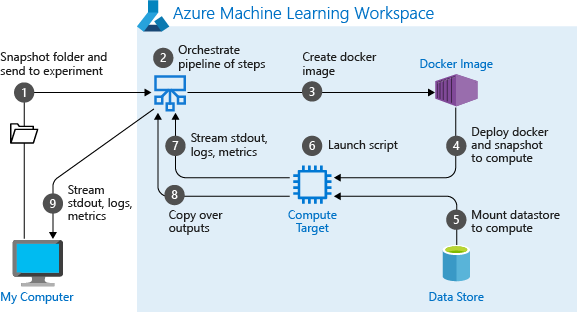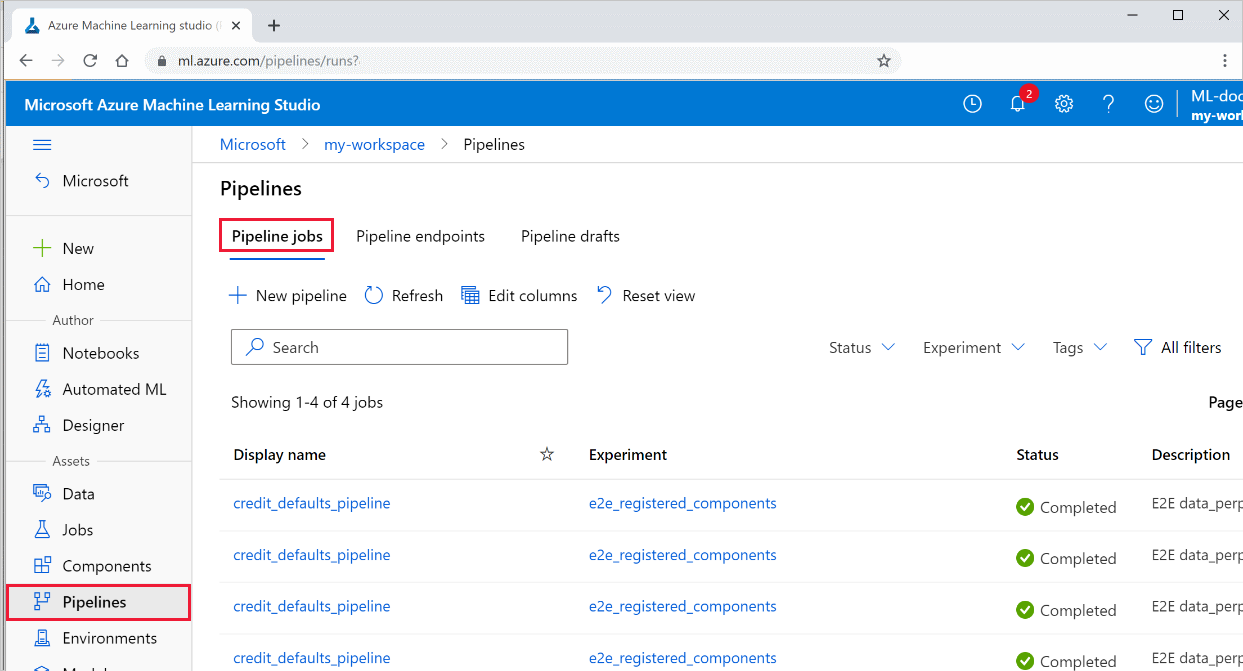# 使用 Azure 机器学习 SDK 创建和运行机器学习管道

Azure 机器学习工作区的成员可以看到创建的 ML 管道。

ML 管道在计算目标上执行（请参阅 Azure 机器学习中的计算目标是什么）。 管道可以在支持的 Azure 存储文档位置读取和写入数据。

## 先决条件

``````import azureml.core
from azureml.core import Workspace, Datastore

ws = Workspace.from_config()
``````

## 设置机器学习资源

• 设置一个数据存储，用于访问管道步骤中所需的数据。

• 配置 `Dataset` 对象，使之指向驻留在数据存储中的或可在数据存储中访问的持久性数据。 为在管道步骤间传递的临时数据配置 `OutputFileDatasetConfig` 对象。

• 设置计算目标，管道步骤将在其上运行。

### 设置数据存储

``````# Default datastore
def_data_store = ws.get_default_datastore()

# Get the blob storage associated with the workspace
def_blob_store = Datastore(ws, "workspaceblobstore")

# Get file storage associated with the workspace
def_file_store = Datastore(ws, "workspacefilestore")

``````

### 使用 `Dataset` 和 `OutputFileDatasetConfig` 对象配置数据

``````from azureml.core import Dataset

my_dataset = Dataset.File.from_files([(def_blob_store, 'train-images/')])
``````

`OutputFileDatasetConfig` 对象返回目录，并且默认将输出写入工作区的默认数据存储。

``````from azureml.data import OutputFileDatasetConfig

output_data1 = OutputFileDatasetConfig(destination = (datastore, 'outputdataset/{run-id}'))
output_data_dataset = output_data1.register_on_complete(name = 'prepared_output_data')

``````

Azure 不会自动删除使用 `OutputFileDatasetConfig` 存储的中间数据。 你应以编程方式在管道运行结束时删除中间数据，使用具有短数据保留策略的数据存储，或定期执行手动清理。

## 设置计算目标

### Azure 机器学习计算

``````from azureml.core.compute import ComputeTarget, AmlCompute

compute_name = "aml-compute"
vm_size = "STANDARD_NC6"
if compute_name in ws.compute_targets:
compute_target = ws.compute_targets[compute_name]
if compute_target and type(compute_target) is AmlCompute:
print('Found compute target: ' + compute_name)
else:
print('Creating a new compute target...')
provisioning_config = AmlCompute.provisioning_configuration(vm_size=vm_size,  # STANDARD_NC6 is GPU-enabled
min_nodes=0,
max_nodes=4)
# create the compute target
compute_target = ComputeTarget.create(
ws, compute_name, provisioning_config)

# Can poll for a minimum number of nodes and for a specific timeout.
# If no min node count is provided it will use the scale settings for the cluster
compute_target.wait_for_completion(
show_output=True, min_node_count=None, timeout_in_minutes=20)

# For a more detailed view of current cluster status, use the 'status' property
print(compute_target.status.serialize())
``````

## 配置训练运行的环境

``````from azureml.core.runconfig import RunConfiguration
from azureml.core import Environment

aml_run_config = RunConfiguration()
# `compute_target` as defined in "Azure Machine Learning compute" section above
aml_run_config.target = compute_target

USE_CURATED_ENV = True
if USE_CURATED_ENV :
curated_environment = Environment.get(workspace=ws, name="AzureML-Tutorial")
aml_run_config.environment = curated_environment
else:
aml_run_config.environment.python.user_managed_dependencies = False

# Add some packages relied on by data prep step
conda_packages=['pandas','scikit-learn'],
pip_packages=['azureml-sdk', 'azureml-dataprep[fuse,pandas]'],
pin_sdk_version=False)
``````

`USE_CURATED_ENV` 更改为 `False` 所采用的路径显示了显式设置依赖项的模式。 在这种情况下，将在资源组内的 Azure 容器注册表中创建和注册新的自定义 Docker 映像（请参阅 Azure 中的专用 Docker 容器注册表简介）。 创建和注册此映像可能需要几分钟的时间。

## 构造管道步骤

``````from azureml.pipeline.steps import PythonScriptStep
dataprep_source_dir = "./dataprep_src"
entry_point = "prepare.py"
# `my_dataset` as defined above
ds_input = my_dataset.as_named_input('input1')

# `output_data1`, `compute_target`, `aml_run_config` as defined above
data_prep_step = PythonScriptStep(
script_name=entry_point,
source_directory=dataprep_source_dir,
compute_target=compute_target,
runconfig=aml_run_config,
allow_reuse=True
)
``````

`arguments` 值指定该步骤的输入和输出。 在上面的示例中，基线数据是 `my_dataset` 数据集。 相应数据会下载到计算资源，因为代码将其指定为 `as_download()`。 脚本 `prepare.py` 执行与手头任务相应的任何数据转换任务，并将数据输出到 `OutputFileDatasetConfig` 类型的 `output_data1`。 有关详细信息，请参阅将数据移入 ML 管道和在 ML 管道之间移动数据的步骤 (Python)。 该步骤将使用配置 `aml_run_config` 在由 `compute_target` 定义的计算机上运行。

``````train_source_dir = "./train_src"
train_entry_point = "train.py"

training_results = OutputFileDatasetConfig(name = "training_results",
destination = def_blob_store)

train_step = PythonScriptStep(
script_name=train_entry_point,
source_directory=train_source_dir,
arguments=["--prepped_data", output_data1.as_input(), "--training_results", training_results],
compute_target=compute_target,
runconfig=aml_run_config,
allow_reuse=True
)
``````

``````# list of steps to run (`compare_step` definition not shown)
compare_models = [data_prep_step, train_step, compare_step]

from azureml.pipeline.core import Pipeline

# Build the pipeline
pipeline1 = Pipeline(workspace=ws, steps=[compare_models])
``````

### 使用数据集

``````dataset_consuming_step = PythonScriptStep(
script_name="iris_train.py",
inputs=[iris_tabular_dataset.as_named_input("iris_data")],
compute_target=compute_target,
source_directory=project_folder
)
``````

``````# iris_train.py
from azureml.core import Run, Dataset

run_context = Run.get_context()
iris_dataset = run_context.input_datasets['iris_data']
dataframe = iris_dataset.to_pandas_dataframe()
``````

`Run.get_context()` 行值得强调。 此函数检索 `Run`（表示当前试验运行）。 在上面的示例中，我们使用它来检索已注册数据集。 `Run` 对象的另一个常见用途是检索试验本身和试验所在的工作区：

``````# Within a PythonScriptStep

ws = Run.get_context().experiment.workspace
``````

## 缓存和重复使用

• 步骤定义期间通过设置 `allow_reuse=False`禁用默认的重复使用步骤运行输出的行为。 在协作环境中使用管道时，“重复使用”非常关键，因为消除不必要的运行可以提高敏捷性。 但是，可以选择禁用重复使用。
• 使用 `pipeline_run = exp.submit(pipeline, regenerate_outputs=True)` 强制对运行中的所有步骤重新生成输出

``````step = PythonScriptStep(name="Hello World",
script_name="hello_world.py",
compute_target=aml_compute,
source_directory=source_directory,
allow_reuse=False,
hash_paths=['hello_world.ipynb'])
``````

## 提交管道

``````from azureml.core import Experiment

# Submit the pipeline to be run
pipeline_run1 = Experiment(ws, 'Compare_Models_Exp').submit(pipeline1)
pipeline_run1.wait_for_completion()
``````

• 将项目快照从与工作区关联的 Blob 存储下载到计算目标。

• 生成对应于管道中每个步骤的 Docker 映像。

• 将每个步骤的 Docker 映像从容器注册表下载到计算目标。

• 配置对 `Dataset``OutputFileDatasetConfig` 对象的访问权限。 在 `as_mount()` 访问模式下，FUSE 用于提供虚拟访问。 如果不支持装载，或者用户将访问权限指定为 `as_upload()`，则改为将数据复制到计算目标。

• 运行在步骤定义中指定的计算目标中的步骤。

• 创建项目，例如日志、stdout 和 stderr、指标以及步骤指定的输出。 然后上传这些项目并将其保存在用户的默认数据存储中。## 对推理时更改的参数使用管道参数

``````from azureml.pipeline.core import PipelineParameter

pipeline_param = PipelineParameter(name="pipeline_arg", default_value="default_val")
train_step = PythonScriptStep(script_name="train.py",
arguments=["--param1", pipeline_param],
target=compute_target,
source_directory=project_folder)
``````

### Python 环境如何处理管道参数

``````aml_run_config = RunConfiguration()
aml_run_config.environment.name = 'MyEnvironment'
aml_run_config.environment.version = '1.0'
``````

## 查看管道的结果

1. 登录到 Azure 机器学习工作室

2. 在左侧，选择“管道”以查看所有管道运行。3. 选择特定的管道以查看运行结果。